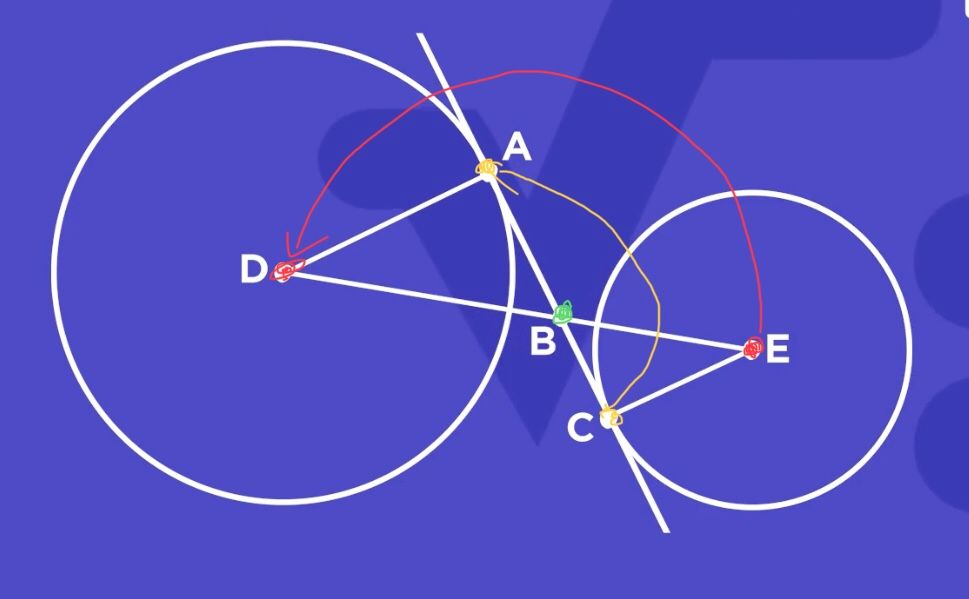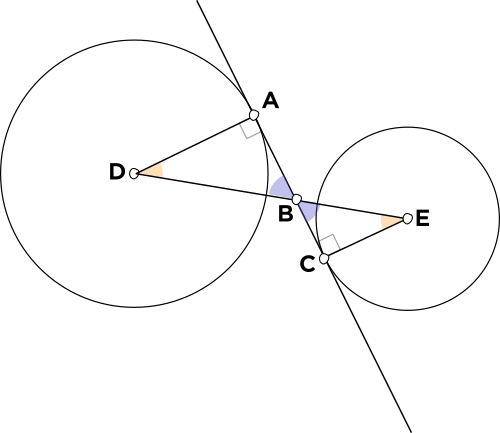# Order

• For the mini question, the correct answer, ii, says AB and BC.However, in this diagram I annotated, AB represents yellow->green and BC represents green->yellow instead.
Even though they are the same length, I think order is quite important and the answer should be changed to something like “AB and CB” or “BA and BC”.

Thanks• @RZ923 Thank you, this is a good suggestion!• @debbie Fixed• ???? huh

• @The-Blade-DancerThe answer choice originally read as "$$\textcolor{blue}{\overline{AB}}$$ and $$\textcolor{blue}{\overline{BC}},$$" and you can see the order of the vertices: in $$\overline{AB},$$ the first vertex, $$A,$$ is at the right angle and the second vertex, $$B,$$ is at the purple angle. In $$\overline{BC},$$ the first vertex, $$B,$$ is at the purple angle, and the second vertex, $$C,$$ is at the right angle.

It would be more consistent to name them starting with the first vertex being at the right angle and the second vertex at the purple angle.

This is what @RZ923 was talking about with regards to the order of the vertices when naming a line segment.

You can also see from the similar triangles $$\bigtriangleup ABD$$ and $$\bigtriangleup CBE$$ that the side $$\overline{AB}$$ corresponds to the side $$\overline{CB}.$$